## Application of Buckingham Pi theorem

The theorem we have stated is a very general one, but by no means limited to Fluid Mechanics. It is used in diversified fields such as Botany and Social Sciences and books and volumes have been written on this topic. But we do not need much theory to be able to apply it. What we will consider is a procedure to use the theorem and arrive at non-dimensional numbers for a given flow.

1. List all the variables that govern the process. These variables should be independent of each other. For example, one should not choose density, gravity and specific weight. Density and Specific Weight should do. For our problem we have F, D, V,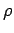and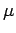. We have n = 5.
2. Mark the Repeating variables. In our case these are D, V andmaking k = 3 .
3. Decide how many non-dimensional numbers are there. For our case we have n - k = 2. Our problem has 2 non-dimensional numbers,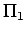and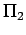.
4. Define the non-dimensional numbers by grouping the variables into n - k groups so that each group has all the repeating variables and one non-repeating variable. Thus for our problem we have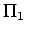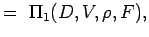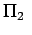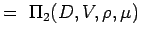(5.2) Let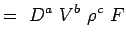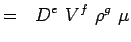(5.3)

5. Now express each variables in terms of its dimensions. Let us use the MLT system according to which the variables for our problems have the following dimensions.

 Variable Dimensions F, Force M L / T2 or M L T-2 D, Diameter: L V, Velocity L/T or LT-1, Density: M/L3 or ML-3, Viscosity ML-1T-1

Substituting these dimensions into Eqn. 5.2, we have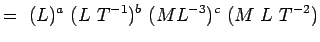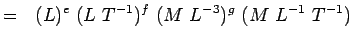(5.4) Or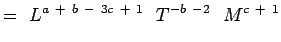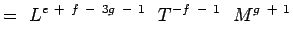(5.5)

Noting thatandare non-dimensional we have,

 a + b - 3c + 1 = 0; -b - 2 = 0, c + 1 = 0 e + f - 3g - 1 = 0; -f - 1 = 0;g + 1 = 0 (5.6)

Solving the equations yields,

 a = -2, b = -2, c = -1 e = -1, f = -1, g = -1 (5.7)

Now our non-dimensional numbers become,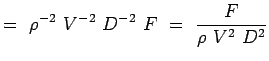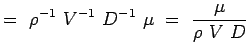(5.8)

Thus we have found the non-dimensional numbers for the flow of interest, namely, drag about a circular cylinder. The functional relationship between the two numbers may be expressed as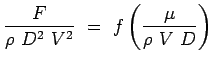(5.9)

But note that the form of term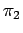we have derived is somewhat different from what we assumed at the beginning. The right hand side of the equation is actually the inverse of Reynolds number! This points to the drawback of the analysis that the exact functional form between the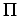numbers cannot be obtained. Any coefficient or index obtained cannot be determined by this analysis. This should be determined by experimentation or by computations. But since the numbers are non-dimensional we can always write,(5.10)

(c) Aerospace, Mechanical & Mechatronic Engg. 2005
University of Sydney